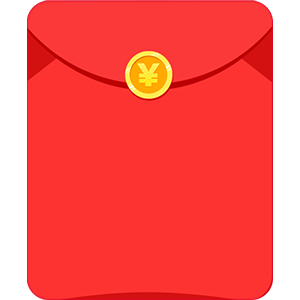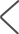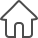甲乙二人在400米的环形跑道上练习长跑，同时以同一起点出发，甲的速度是每秒6米，乙的速度是每秒4米，乙跑几圈后甲可超过乙一圈？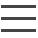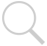×

9-99元的随机现金红包`甲乙二人在400米的环形跑道上练习长跑，同时以同一起点出发，甲的速度是每秒6米，乙的速度是每秒4米，乙跑几圈后甲可超过乙一圈？`
`8是哪里来的`

```解设两人同时同方向出发X秒后第一次相遇
6x-4X=400
2X=400
X=200(秒）
4X=1200 1200÷400=3（圈）
4X=1600 1600÷400=4（圈）

```
```解设两人同时同方向出发X秒后第一次相遇
8X-6X=400
2X=400
X=200(秒）
6X=1200 1200÷400=3（圈）
8X=1600 1600÷400=4（圈）
答同时出发200秒后相遇
此时甲跑了4圈，乙跑了3圈。```
```解设两人同时同方向出发X秒后第一次相遇
8X-6X=400
2X=400
X=200(秒）
6X=1200  1200÷400=3（圈）
8X=1600    1600÷400=4（圈）

```
```解设两人同时同方向出发X秒后第一次相遇
8X-6X=400
2X=400
X=200(秒）
6X=1200  1200÷400=3（圈）
8X=1600    1600÷400=4（圈）